Ch 2. Fluid Statics Multimedia Engineering Fluids PressureVariation PressureMeasurement HydrostaticForce(Plane) HydrostaticForce(Curved) Buoyancy
 Chapter 1. Basics 2. Fluid Statics 3. Kinematics 4. Laws (Integral) 5. Laws (Diff.) 6. Modeling/Similitude 7. Inviscid 8. Viscous 9. External Flow 10. Open-Channel Appendix Basic Math Units Basic Equations Water/Air Tables Sections Search eBooks Dynamics Fluids Math Mechanics Statics Thermodynamics Author(s): Chean Chin Ngo Kurt Gramoll ©Kurt GramollFLUID MECHANICS - THEORY

In this section, attention is focused on the discussion of a fluid at rest or moving in a way such that there is no presence of shearing stresses. The variations of pressure with direction and depth in a fluid will be examined.

Pressure at a Point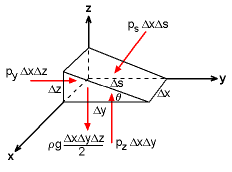Force Balance on a
Triangular Element

Consider a small triangular wedge of fluid taken from an arbitrary location within a fluid mass to determine the variation of pressure with direction. With the absence of shear stresses, the only forces acting on the fluid element are the normal and gravitational forces. Note that the forces in the x direction are not shown for clarity. According to Newton’s second law, the forces in the y and z directions can be summed as

y-direction:
py ΔxΔz - ps ΔxΔs sinθ = ρ (ΔxΔyΔz/2) ay

z-direction:
pz ΔxΔy - ps ΔxΔs cosθ - ρ (ΔxΔyΔz/2) g
= ρ (ΔxΔyΔz/2) az

Since Δz = Δs sinθ and Δy = Δs cosθ, the above equations reduce to

py - ps = ρ(Δy/2) ay

pz - ps = ρ (Δz/2) (g + az)

By shrinking the fluid element to a point, i.e., Δx, Δy, and Δz approach zero, it can be seen that

py = pz = ps

These results are known as Pascal’s law, which states that the pressure at a point in a static fluid is independent of direction. In other words, pressure is a scalar for fluids.

Pressure Field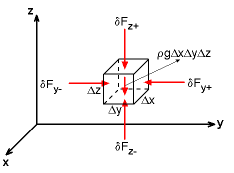Force Balance on a
Rectangular Element

Next, consider a small rectangular fluid element taken from an arbitrary location within a fluid mass. Let the pressure at the center of the element be p. Then, use the first order Taylor series expansion to determine the forces at the faces of the fluid element (forces in the x-direction are not shown for clarity). For the force at the x+ face: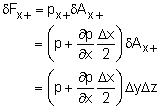Similarly, the forces at other faces can be written as: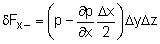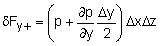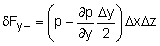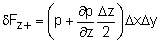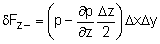By assuming the only body force acting on the fluid element is due to gravity (i.e., the weight of the fluid element) and the absence of shearing forces (i.e., inviscid assumption), then applying Newton’s second law in the x-direction and yields

x-direction: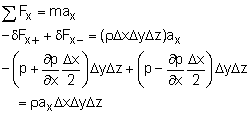Similarly, according to Newton's second law, the force balances in the y- and z- directions are given by

y-direction: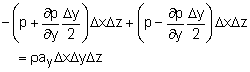z-direction: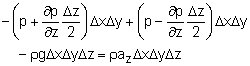The above equations can be simplified to obtain

x-direction: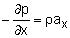y-direction: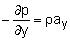z-direction: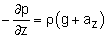These equations can be recast into vector notation: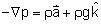where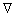is the gradient operator and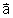is the acceleration vector of the fluid element.

Pressure Variation in a Static Fluid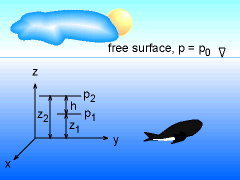Derivation of Hydrostatic
Pressure Distribution

For a fluid at rest (i.e.,= 0), we have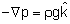or in scalar form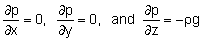Hence, it can be concluded that p varies only in the z-direction and is not a function of x and y, giving

dp/dz = -ρg

The above equation determines how pressure changes with elevation for a fluid at rest. Integration can be performed to obtain the changes in pressure once the variation of gravity and fluid density with elevation is known. For most practical engineering applications, the variation of g is negligible over the change in height. This gives,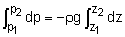p1 - p2 = ρg (z2 - z1)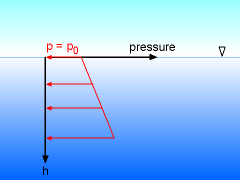Hydrostatic Pressure Distribution
in a Static Fluid

Thus, pressure increases with depth in a linear fashion. This type of pressure distribution is called hydrostatic. We can also rearrange the above equation to yield an expression for the pressure head as follows:

h = (p1 - p2) / ρg

Physically, the pressure head represents the height of a homogeneous fluid column required to produce a pressure difference of (p1 - p2). The term ρg is often written as γ (specific weight) in civil engineering applications.

Practice Homework and Test problems now available in the 'Eng Fluids' mobile app
Includes over 250 problems with complete detailed solutions.
Available now at the Google Play Store and Apple App Store.